The Rocscience International Conference 2021 Proceedings are now available. Read Now

# Water Parameters

For each material in the Define Material Properties dialog, Water Parameters can be defined, which determine the pore pressure calculation for each material. The Water Parameters depend on the Groundwater Method chosen in the Project Settings dialog.

NOTE: for materials using one of the following Strength Type models:

• Undrained
• No Strength
• Infinite Strength

Water Parameters are not applicable, and are disabled.

Water Surfaces

If the Groundwater Method in Project Settings is Water Surfaces, then the following Water Parameters will apply:

Water Surface

The user must choose the Water Surface (Water Table or Piezo Line ) which corresponds to the material type (soil region) they are defining. Only existing Water Surfaces will appear in the list. Piezo Lines are identified by an ID number. The user may also choose None (this is the default selection) if no Water Surface is associated with a given material. Pore pressure will be zero for a material, if Water Surface = None.

## Hu Coefficient

The Hu Coefficient as defined in Slide2 is simply a factor between 0 and 1, by which the VERTICAL distance from a point in the soil (e.g. the center of a slice base) to a Water Surface (either a Water Table or Piezo Line) is multiplied to obtain the pressure head. The Hu Coefficient is used to calculate the pore pressure as follows: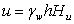where: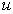= pore pressure= the Pore Fluid Unit Weight (entered in the Project Settings dialog)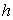= the vertical distance from the base of a slice to a Water Surface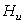= the Hu coefficient for the soil type (either user defined or Auto, see below)

NOTE:

• If the distance h is negative, (i.e. Water Surface is below the base of a slice) then the pore pressure is set to zero.
• If a Water Surface is not defined above a given slice, then the safety factor calculation for that particular slip surface will not proceed, and an error message will be written to the file. It is up to the user to ensure that Water Surfaces span all of the required soil regions.
1. There are two ways of defining the Hu Coefficient - Auto or Custom.

## Custom Hu

With the Custom option, the user can enter their own value for Hu. A value between 0 and 1 must be specified. For example:

• Hu = 1 would indicate hydrostatic conditions. This can be used where the Water Surface is horizontal. Where the Water Surface is inclined, setting Hu = 1 will provide a conservative (low) estimate of the safety factor, since in general this will overestimate the true pore pressure. In most cases, the user will simply set Hu = 1, because this represents the worst case scenario (maximum pore pressure) assuming steady state conditions.
• Hu = 0 would indicate a dry soil. Pore pressure will be zero. Setting Hu = 0 can be used to turn "off" the pore pressure for a material, although this can also be achieved by setting Water Surface = None.
• Intermediate values of Hu can be used to simulate head loss due to seepage. This would be applicable where the Water Surface is inclined. The user could create a separate material region for each segment of the Water Surface which is inclined, and enter Hu values less than 1. However, the Auto Hu option, described below, can be used to automatically account for the inclination of the Water Surface.
2. NOTE: if you are using Piezometric Lines, you should, strictly speaking, use the Custom Hu option, with Hu = 1. This is because a Piezometric Line is usually a direct representation of the pressure head, for a specific slip surface. However, in Slide2, the user may decide how to apply the Hu Coefficient, for any type of Water Surface (Water Table or Piezometric Lines).

## Auto Hu

With the Auto Hu option, Slide2 will automatically calculate a value of Hu, based on the inclination (angle) of the Water Surface, above any given point. This is based on the assumption that the equipotential line which passes through the center of a slice base, is a straight line, between the slice base and the Water Surface (strictly applicable for an infinite slope case). This is illustrated below.

•= the inclination of the Water Surface (above a given point)
•= VERTICAL distance, from center of slice base, to Water Surface

## Automatic Calculation of Hu coefficient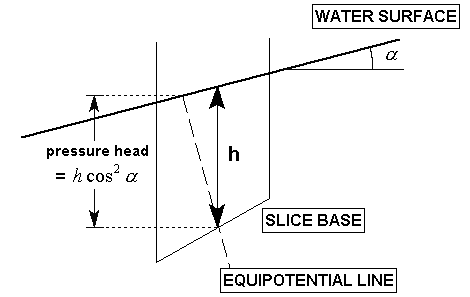The Auto Hu option is a useful method of estimating pore pressures, based on the inclination of a Water Surface. In the absence of more accurate data (e.g. Seepage Analysis results), this is a simple but useful method of approximating head loss due to seepage.

## Ru Coefficient

If the Groundwater Method = Ru Coefficient in Project Settings, then the following Water Parameters will apply:

## Ru Value

An Ru coefficient between 0 and 1 must be specified. The Ru coefficient used in Slide2 is the one widely used, which simply models the pore pressure as a fraction of the vertical earth pressure for each slice.

NOTE: the calculation of the vertical earth pressure includes the weight of ponded water, if a material is submerged under water. However, the vertical pressure does NOT include forces due to external loads (e.g. line, distributed or seismic loads).

If one soil type has regions of differing Ru values, then a different material will have to be defined for each different Ru value. Appropriate Material Boundaries will have to be added to the model, in order to define the different soil regions.

## Using Ru with Water Surfaces or Grids

It is possible to use the Ru method of pore pressure calculation, in conjunction with either Water Surfaces or Water Pressure grids.

• If the Groundwater Method (in Project Settings) = Water Surfaces, and Water Surfaces = None (in Define Materials), you will be able to enter an Ru value for the material.
• If the Groundwater Method (in Project Settings) = one of the 3 Water Pressure Grid methods, and Grid = Off (in Define Materials), you will be able to enter an Ru value for the material.

For each material, only one method of pore pressure calculation can be used. For example, if you specify a Water Surface for a material (e.g. Water Surface = Water Table), then an Ru value cannot be used for that material. If you are using an Ru value for a material, then you cannot specify a Water Surface for that material (i.e. Water Surface = None). You cannot simultaneously use more than one method of (initial) pore pressure calculation, for a single material.

## Pore Pressure Grids

If the Groundwater Method in Project Settings is one of the three Grid options (Total Head, Pressure Head or Pore Pressure), then the following Water Parameters will apply:

## Grid On/Off

The effect of a pore pressure grid can be turned ON or OFF for a given material. If toggled OFF, then pore pressure will be set to zero for that material, and no pore pressure calculations will be done for that material. Default is ON.

## Finite Element Analysis

If the Groundwater Method = Steady State FEA in Project Settings, then Water Parameters are NOT defined in the Define Material Properties dialog, because the pore pressures are calculated by the finite element Groundwater Analysis. However, you will be able to enter Unsaturated Shear Strength parameters (Phib and Air Entry Value) which define the rate of increase of shear strength, due to negative pore pressures in the unsaturated zone. See the Unsaturated Shear Strength topic for details.

## Initial Pore Pressure / Excess Pore Pressure

If you are using the B-bar method to calculate excess pore pressure due to undrained loading, then the Water Parameters, as described above, will define the Initial Pore Pressure for a material. Excess pore pressure is calculated using the B-bar method (see the Excess Pore Pressure topic for details). The final pore pressure used in the stability analysis is the sum of the Initial Pore Pressure + Excess Pore Pressure.

## Specify alternate strength type above water surface

This option allows you to specify different strength types above and below the assigned water surface for any material, by selecting a different material be used for the strength above the water table. See the Strength Above Water Surface topic for more information.